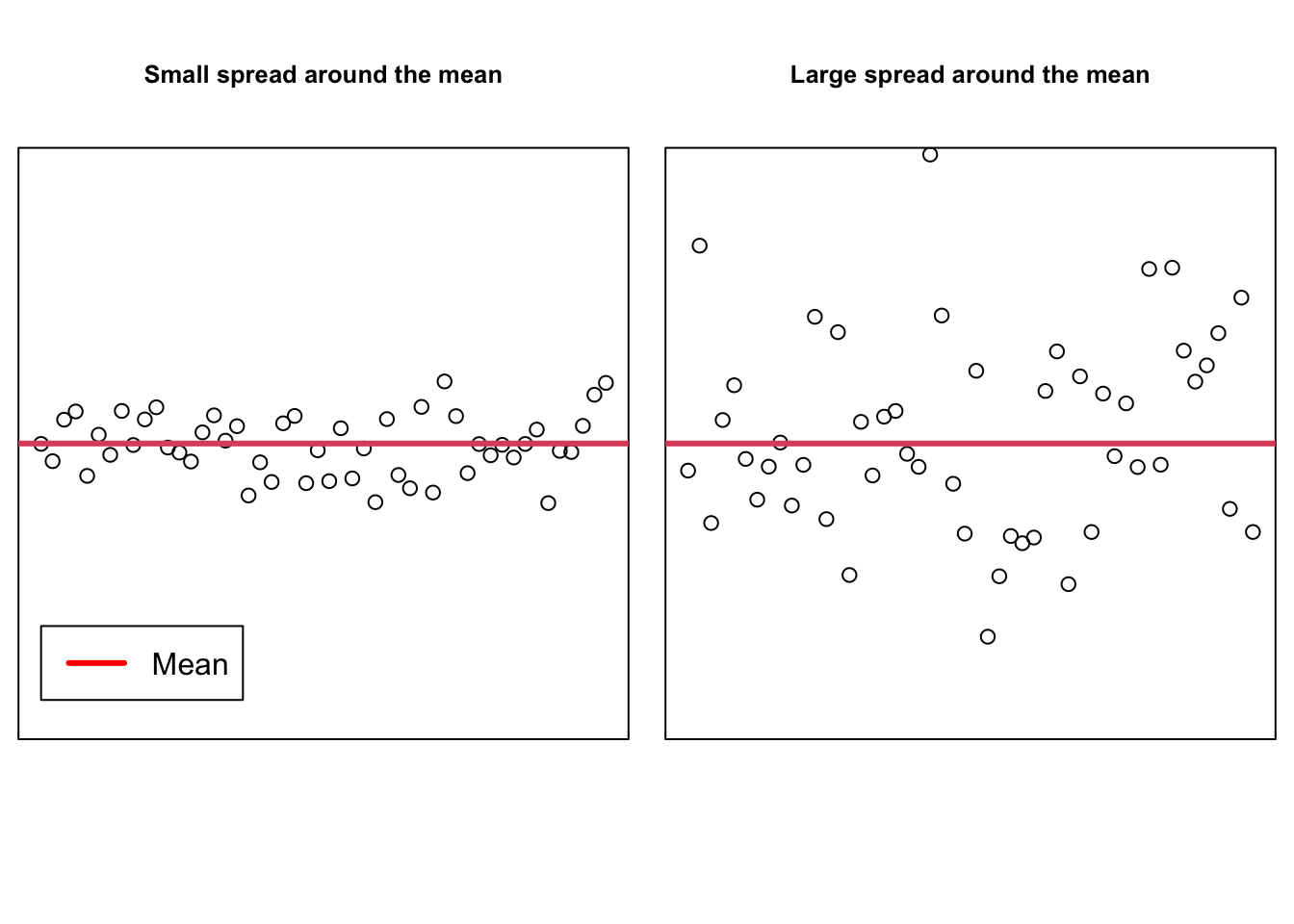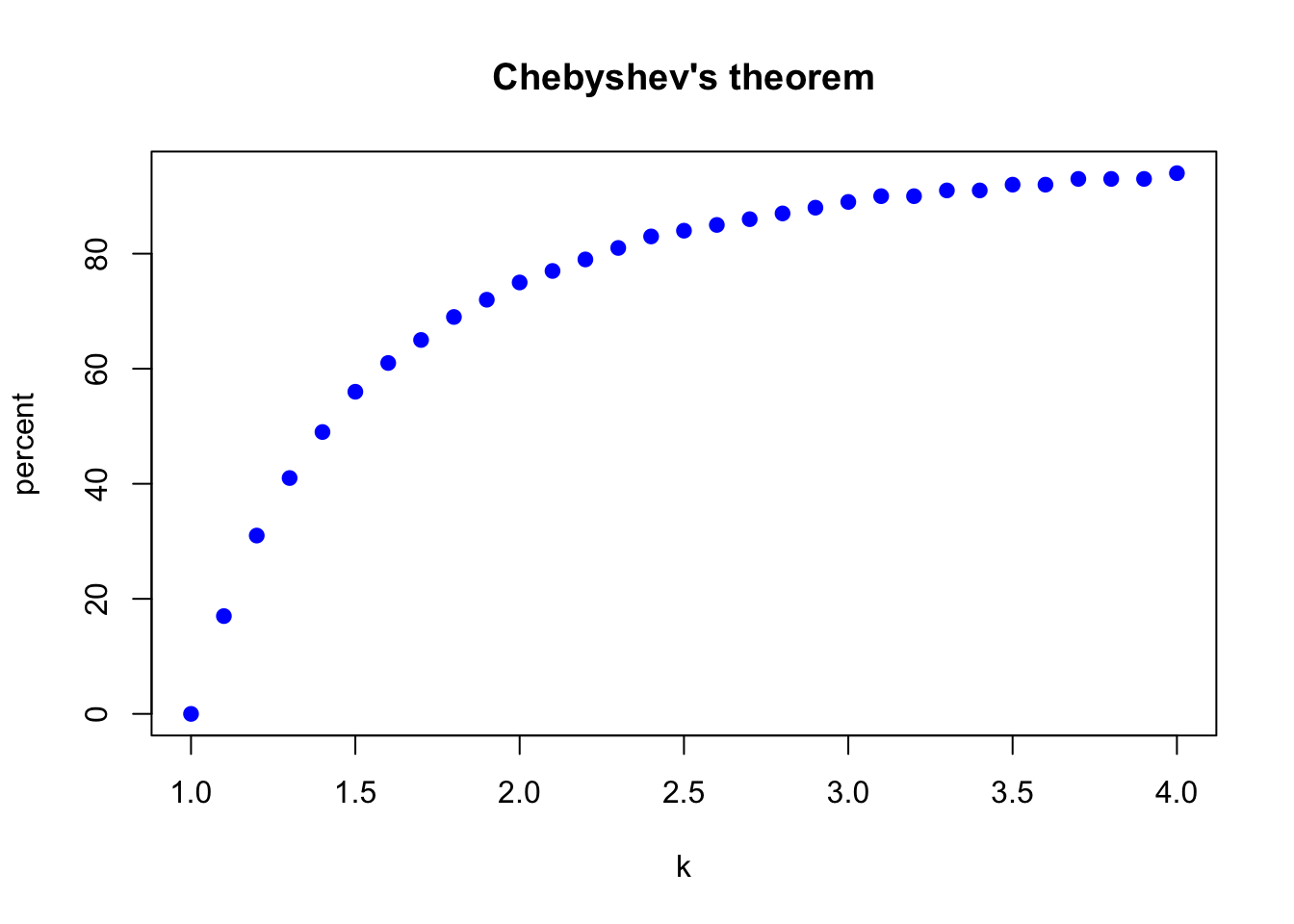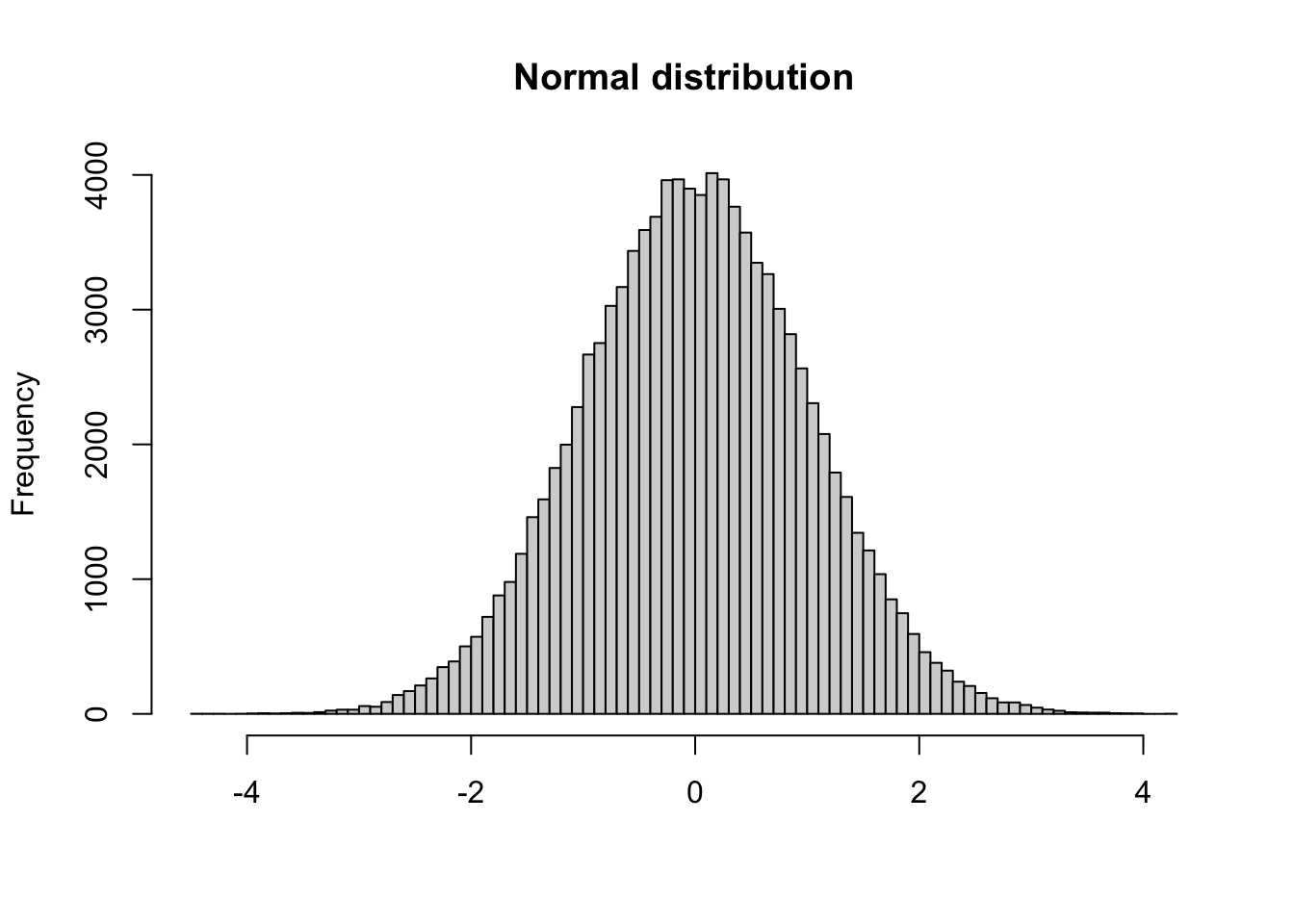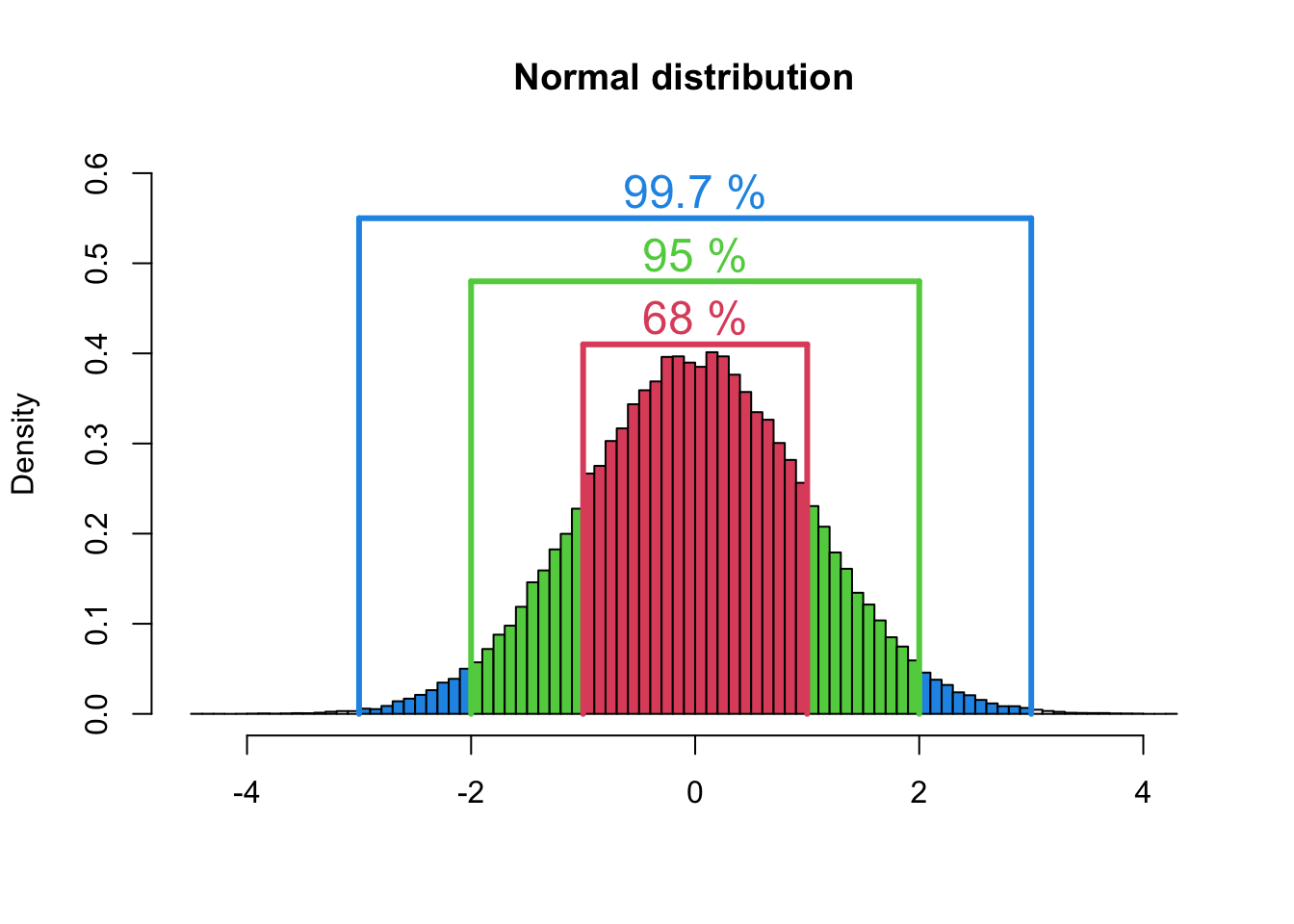The variance is the mean squared deviation from the mean. The variance for population data is denoted by $$\sigma^2$$ (read as sigma squared) and the variance calculated for sample data is denoted by $$s^2$$.

$\sigma^2 = \frac{\sum_{i=1}^n (x_i-\mu)^2}{N}$

and

$s^2 = \frac{\sum_{i=1}^n (x_i-\bar x)^2}{n-1}$

where $$\sigma^2$$ is the population variance and $$s^2$$ is the sample variance. The quantity $$x_i-\mu$$ or $$x_i-\bar x$$ in the above formulas is called the deviation of the $$x_i$$ value ($$x_1, x_2,...,x_n$$) from the mean (Mann 2012).

The standard deviation is the most-used measure of dispersion. The value of the standard deviation tells us how closely the values of a data set are clustered around the mean. In general, a lower value of the standard deviation indicates that the values of the data set are spread over a relatively smaller range around the mean. In contrast, a larger value of the standard deviation for a data set indicates that the values of that data set are spread over a relatively larger range around the mean (Mann 2012).The standard deviation is obtained by taking the square root of the variance. Consequently, the standard deviation calculated for population data is denoted by $$\sigma$$ and the standard deviation calculated for sample data is denoted by $$s$$.

$\sigma = \sqrt{\frac{\sum_{i=1}^N (x_i-\mu)^2}{N}}$

and

$s = \sqrt{\frac{\sum_{i=1}^n (x_i-\bar x)^2}{n-1}}$

where $$\sigma$$ is the standard deviation of the population and $$s$$ is the standard deviation of the sample.

As an exercise we compute the mean, the median, the variance and the standard deviation for some numerical variables of interest in the students data set, and present them in a nice format.

students <- read.csv("https://userpage.fu-berlin.de/soga/data/raw-data/students.csv")
quant_vars <- c("name", "age", "nc.score", "height", "weight")
students_quant <- students[quant_vars]
head(students_quant, 10)
##                    name age nc.score height weight
## 1   Gonzales, Christina  19     1.91    160   64.8
## 2        Lozano, T'Hani  19     1.56    172   73.0
## 3        Williams, Hanh  22     1.24    168   70.6
## 4           Nem, Denzel  19     1.37    183   79.7
## 5       Powell, Heather  21     1.46    175   71.4
## 6        Perez, Jadrian  19     1.34    189   85.8
## 7         Clardy, Anita  21     1.11    156   65.9
## 8  Allen, Rebecca Marie  21     2.03    167   65.7
## 9         Tracy, Robert  18     1.29    195   94.4
## 10       Nimmons, Laura  18     1.19    165   66.0
# mean
students_quant_mean <- apply(students_quant[, !(colnames(students_quant) == "name")], 2, mean)
# median
students_quant_median <- apply(students_quant[, !(colnames(students_quant) == "name")], 2, median)
# variance
students_quant_var <- apply(students_quant[, !(colnames(students_quant) == "name")], 2, var)
# standard deviation
students_quant_sd <- apply(students_quant[, !(colnames(students_quant) == "name")], 2, sd)
# concatenate the vectors and round to 2 digits
students_quant_stats <- round(cbind(
students_quant_mean,
students_quant_median,
students_quant_var,
students_quant_sd
), 2)
# rename column names
colnames(students_quant_stats) <- c("mean", "median", "variance", "standard deviation")
students_quant_stats
##            mean median variance standard deviation
## age       22.54  21.00    36.79               6.07
## nc.score   2.17   2.04     0.66               0.81
## height   171.38 171.00   122.71              11.08
## weight    73.00  71.80    74.57               8.64

#### Use of the Standard Deviation

By using the mean and standard deviation we can find the proportion or percentage of the total observations that fall within a given interval around the mean.

##### Chebyshev’s Theorem

Chebyshev’s theorem gives a lower bound for the area under a curve between two points that are on opposite sides of the mean and at the same distance from the mean.

For any number $$k$$ greater than 1, at least $$(1-1/k^2)*100$$ % of the data values lie within $$k$$ standard deviations of the mean.

Let us use R to gain some intuition for Chebyshev’s theorem.

k <- seq(1, 4, by = 0.1)
auc <- 1 - (1 / k^2)
auc_percent <- round(auc * 100)
cbind(k, auc_percent)
##         k auc_percent
##  [1,] 1.0           0
##  [2,] 1.1          17
##  [3,] 1.2          31
##  [4,] 1.3          41
##  [5,] 1.4          49
##  [6,] 1.5          56
##  [7,] 1.6          61
##  [8,] 1.7          65
##  [9,] 1.8          69
## [10,] 1.9          72
## [11,] 2.0          75
## [12,] 2.1          77
## [13,] 2.2          79
## [14,] 2.3          81
## [15,] 2.4          83
## [16,] 2.5          84
## [17,] 2.6          85
## [18,] 2.7          86
## [19,] 2.8          87
## [20,] 2.9          88
## [21,] 3.0          89
## [22,] 3.1          90
## [23,] 3.2          90
## [24,] 3.3          91
## [25,] 3.4          91
## [26,] 3.5          92
## [27,] 3.6          92
## [28,] 3.7          93
## [29,] 3.8          93
## [30,] 3.9          93
## [31,] 4.0          94

To put it in words: Let us pick a value for $$k$$: $$k= 2$$. This means that at least 75% of the data values lie within 2 standard deviations of the mean.

Let us plot Chebyshev’s theorem with R:

plot(k,
auc_percent,
col = "blue",
pch = 19,
xlab = "k",
ylab = "percent",
main = "Chebyshev's theorem"
)The theorem applies to both sample and population data. Note that Chebyshev’s theorem is applicable to a distribution of any shape. However, Chebyshev’s theorem can be used only for $$k>1$$. This is so because when $$k = 1$$, the value of $$(1-1/k^2)$$ is zero, and when $$k<1$$, the value of $$(1-1/k^2)$$ is negative (Mann 2012).

##### Empirical Rule

While Chebyshev’s theorem is applicable to any kind of distribution, the empirical rule applies only to a specific type of distribution called a bell-shaped distribution or normal distribution. There are 3 rules:

For a bell-shaped distribution, approximately

1. 68% of the observations lie within one standard deviation of the mean.
2. 95% of the observations lie within two standard deviations of the mean.
3. 99.7% of the observations lie within three standard deviations of the mean.

Since we have sufficient coding abilities by now, we will try to test if the three rules are valid. (1) First, we will explore the rnorm function in R to generate normally distributed data and (2) second, we will go back to our students data set and validate those rules on that data set.

The normal distribution belongs to the family of continuous distributions. In R there are a lot of probability distributions available (here). To generate data from a normal distribution one may use the rnorm() function, which is a random variable generator for the normal distribution.

We can sample n values from a normal distribution with a given mean (default is 0) and standard deviation (default is 1) using the rnorm() function: rnorm(n=1, mean=0, sd=1). Let us give it a try:

rnorm(n = 1, mean = 0, sd = 1)
##  1.257319
rnorm(n = 1, mean = 0, sd = 1)
##  0.8989994
rnorm(n = 1, mean = 0, sd = 1)
##  0.1279233
rnorm(n = 1, mean = 0, sd = 1)
##  -1.83574

As we can see, the rnorm() function returns (pseudo-)random numbers. We can fairly easily ask the function to draw hundreds or thousands or even more (pseudo-)random numbers:

rnorm(n = 10, mean = 0, sd = 1)
##    0.16461710  0.03458354  0.12142757 -2.19208749  0.25712025 -0.91833172
##   -2.19976991  0.71588844  0.50140881 -1.23618893
rnorm(n = 100, mean = 0, sd = 1)
##     0.5222221012  1.8607926019 -1.5206945718  2.2183712898 -1.2065822744
##     1.2704146564  0.3580718129  0.4689079940 -0.9553892336 -1.3772157146
##   -0.9084666865  0.3099182591  1.2758022662 -0.1985781772  0.7430670468
##   -0.5463838541  0.7213202099 -0.9364125367 -0.6732683741 -0.1496904261
##   -1.8482336532  0.5178397524  0.7525924751 -0.7070632410  0.0313032430
##   -0.0418636490 -1.2666821202 -0.6196303182 -0.2706374512  0.1525131454
##   -1.3060081729 -0.5469122355 -0.5638126372  1.2635819477  1.0648388204
##    0.8371715586  1.1886034962  0.4173571106 -1.0422001302  0.0137474995
##   -0.1823683734 -0.2505076650 -0.6676213745 -0.8767859190 -0.2920202702
##   -0.0232080467 -1.3524766434  1.8269318873 -2.0370244554 -0.5108222951
##   -0.6797826422  0.8084150623  0.4122810100  0.4589805029 -0.2023382148
##   -1.6122332159  0.0005210122  0.2118302938 -1.1004505064  0.1680075469
##   -1.4475047260  0.1033762807 -0.9551496346  0.1437890641  1.0858265253
##   -1.4592614057  0.2470932102  0.2850866672  0.1878534330 -1.4291918313
##    0.3359312228  1.1699267438  0.3181108174  1.7275518252 -0.1066412189
##    1.0896644545  0.5443042711  1.2757819613 -1.1372746856 -0.4807322849
##    0.8842538307  0.7419005173  0.4233802532 -0.4236645169  0.1803395424
##   -0.8444667907  0.4975258473 -1.1653242515 -1.0665594535  0.2178598555
##    1.3336681439  0.6751259279  1.1904379567  0.8275887122 -0.1600961894
##   -0.5458564644 -0.7350187300  0.2060333516 -0.3098440346  0.0355555847
y_norm <- rnorm(n = 100000, mean = 0, sd = 1)

If we plot a histogram of those numbers, we see the eponymous bell shaped distribution.

hist(y_norm, breaks = 100, main = "Normal distribution", xlab = "")We already know the mean and the standard deviation of the y_norm vector, as we explicitly called the function rnorm() with mean = 0 and sd = 1. So, we just have to count those numbers of the y_norm vector bigger than 1, and respectively smaller than -1, bigger than 2, respectively -2, and 3, respectively -3, and relate them to the length of the vector, in our case 100,000, to validate the three rules claimed above.

sd1 <- sum(y_norm > -1 & y_norm < 1) / length(y_norm) * 100
sd2 <- sum(y_norm > -2 & y_norm < 2) / length(y_norm) * 100
sd3 <- sum(y_norm > -3 & y_norm < 3) / length(y_norm) * 100

cbind(c("1sd", "2sd", "3sd"), c(sd1, sd2, sd3))
##      [,1]  [,2]
## [1,] "1sd" "68.324"
## [2,] "2sd" "95.382"
## [3,] "3sd" "99.712"

Perfect match! The three empirical rules are obviously valid. To visualize our findings we re-plot the histogram and add some annotations. Please note that in the hist() function we set the argument freq = F, which is the same as freq = FALSE. As a consequence, the resulting histogram does not show counts on the y-axis anymore, but the density values (normalized count divided by bin width), which means that the bar areas sum to 1.

h <- hist(y_norm, breaks = 100, plot = F)
cuts <- cut(h\$breaks, c(-Inf, -3, -2, -1, 1, 2, 3, Inf), right = F) # right = False;
# sets intervals to be open on the right closed on the left
plot(h,
col = rep(c("white", "4", "3", "2", "3", "4", "white"))[cuts],
main = "Normal distribution",
xlab = "",
freq = F,
ylim = c(0, 0.6)
)

lwd <- 3
# horizontal lines
lines(x = c(2, -2), y = c(0.48, 0.48), type = "l", col = 3, lwd = lwd)
lines(x = c(3, -3), y = c(0.55, 0.55), type = "l", col = 4, lwd = lwd)
lines(x = c(1, -1), y = c(0.41, 0.41), type = "l", col = 2, lwd = lwd)
# vertical lines
lines(x = c(1, 1), y = c(0, 0.41), type = "l", col = 2, lwd = lwd)
lines(x = c(-1, -1), y = c(0, 0.41), type = "l", col = 2, lwd = lwd)
lines(x = c(2, 2), y = c(0, 0.48), type = "l", col = 3, lwd = lwd)
lines(x = c(-2, -2), y = c(0, 0.48), type = "l", col = 3, lwd = lwd)
lines(x = c(3, 3), y = c(0, 0.55), type = "l", col = 4, lwd = lwd)
lines(x = c(-3, -3), y = c(0, 0.55), type = "l", col = 4, lwd = lwd)
# text
text(0, 0.44, "68 %", cex = 1.5, col = 2)
text(0, 0.51, "95 %", cex = 1.5, col = 3)
text(0, 0.58, "99.7 %", cex = 1.5, col = 4)Well, now let us work on our 2nd task: Validate the three empirical rules on the students data set. For this, we have to check whether any of the numeric variables in the students data set are normally distributed. We start by extracting numeric variables of interest from the students data set. Then we check the data set by calling the function head().

cont_vars <- c("age", "nc.score", "height", "weight", "score1", "score2", "salary")
students_quant <- students[, cont_vars]
head(students_quant, 10)
##    age nc.score height weight score1 score2 salary
## 1   19     1.91    160   64.8     NA     NA     NA
## 2   19     1.56    172   73.0     NA     NA     NA
## 3   22     1.24    168   70.6     45     46     NA
## 4   19     1.37    183   79.7     NA     NA     NA
## 5   21     1.46    175   71.4     NA     NA     NA
## 6   19     1.34    189   85.8     NA     NA     NA
## 7   21     1.11    156   65.9     NA     NA     NA
## 8   21     2.03    167   65.7     58     62     NA
## 9   18     1.29    195   94.4     57     67     NA
## 10  18     1.19    165   66.0     NA     NA     NA

To get an overview of the shape of the distribution of each particular variable, we apply the histogram() function of the lattice package. If the lattice package is not yet installed on your computer, type install.packages("lattice")in your console. The coding is a little bit different than for standard histograms.

library(lattice)
histogram(~ height + age + weight + nc.score + score1 + score2 + salary,
breaks = 50,
type = "density",
xlab = "",
ylab = "density",
layout = c(4, 2),
scales = list(relation = "free"),
col = "black",
data = students_quant
)